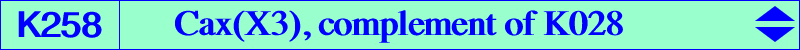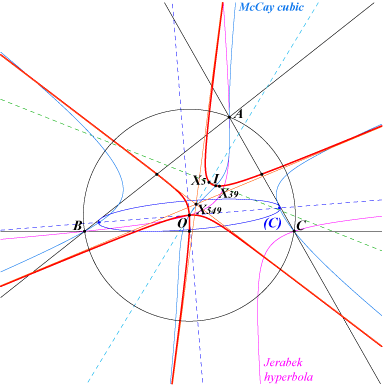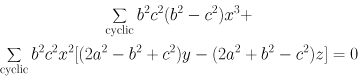X(1), X(3), X(5), X(39), X(2140), X(14823), X(15345), X(47055) midpoints of ABC excenters points at infinity of the McCay cubic Va, Vb, Vc mentioned in the Neuberg cubic page. See also table 16 and table 18. midpoints of the cevians of O projections of O on the six bisectors of ABCThe locus of the perspector P of inscribed conics such that one axis passes through a fixed point Q is in general a sextic which is the isogonal transform of a cubic. This cubic is a circum-cubic if and only if Q is the circumcenter O of ABC. In this case, the sextic decomposes into the line at infinity, the Steiner circum-ellipse and K257. The locus of the centers of the corresponding inscribed conics is K258. See a generalization at CL047, article Cax(F), and also K858 = Cax(X4). K258 is a nodal K60+, i.e. an equilateral cubic with concurring asymptotes at X(549), the midpoint of GO. These asymptotes are parallel to those of the McCay cubic. The node is O with nodal tangents parallel to the asymptotes of the Jerabek hyperbola. These nodal tangents are in fact the axes of (C), the inscribed conic with center O, perspector X(69). K258 meets BC at the midpoint Ma and two other points symmetric with respect to the cevian point of O. These two points are real if and only if the angle A is acute. K258 is the complement of K028, the Musselman (third) cubic hence K258 is a psK with respect to the medial triangle. K258 is the Laplacian of the quintic Q091 i.e. the locus of points whose polar conic in the quintic is a rectangular hyperbola. *** Another description is the following : if P and P' are two antipodes on the circumcircle, the Simson lines of P and P' concur at X on the nine point circle and meet the line OPP' at two points Q, Q' on the quintic Q011. The circle with diameter QQ' is tangent at X to the nine point circle and the locus of its center is K258. K077, K078, K100, K258 are members of a same pencil of cubics generated by the McCay cubic K003 and the decomposed cubic which is the union of the Stammler hyperbola and the line at infinity. See Table 63. See other properties and a generalization in the paper "Another kind of Lemoine cubics" where K258 is L(X3). See also K003, locus property 18.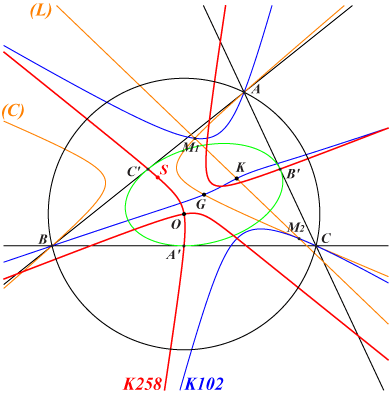Consider a variable line (L) passing through the Lemoine point K and the circum-conic (C) which is its isogonal transform hence passing through G. The center Ω of (C) lies on the Steiner inellipse. (L) and (C) intersect at M1, M2 on the Grebe cubic K102. Let S be the pole of (L) with respect to (C) i.e. the intersection of the tangents at M1, M2 to (C). When (L) varies, this point S lies on K258 and the line SΩ always contains X(39). S coincides with the node O when (L) is parallel to the asymptotes of the Jerabek hyperbola and/or to the axes of the inconic with center K. S coincide with X(5), X(39), X(2140) when (L) passes through X(3), X(2), X(31) respectively.More generally, when K is replaced with a point P, we obtain a cubic K(P) which is very similar to K258. Indeed, K(P) is also a nodal cubic passing through : • the 4 in/excenters, • the vertices A', B', C' of the cevian triangle of the isogonal conjugate P* of P, • Q = P*/P (Ceva conjugate) which is the node, • R = crosspoint of P, P* obtained when (L) passes through P*. Note that the center Ω of (C) lies on the bicevian conic C(G, P*). The affine mapping that sends A'B'C' onto ABC transforms K(P) into a psK – with respect to ABC – analogous to K028.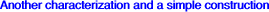Let (L) be a variable line passing through the circumcenter O with infinite point P. Let (H) be the rectangular diagonal hyperbola passing through P and the in/excenters. When (L) rotates around O, (L) and (H) meet at P and another point M on K258.